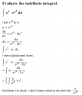# Stuck in Evaluating an Indefinite Integral

• whatisphysics
In summary, eumyang attempted to use substitution to solve for the integral but had an issue before. They then tried using u = x^8 and got the same result as before. They then tried using u = x^8 and got the same result as before.f

## Homework Statement

Evaluate the indefinite integral.

## Homework Equations

I tried to use substitution.

## The Attempt at a Solution

I'm not so good with using the LaTex codes...so I attached a file I made in Word.

#### Attachments

•question4.PNG
6.1 KB · Views: 1,382
Last edited:
Try using u = x^8

Try using u = x^8

The x^8 from e^(x^8) or x^7?

But besides a poor choice of u in your original solution you don't carry out the integral properly

With my choice of u (u = x^8) compute du/dx and show me the new integral

Okay, I've now ended up with

\\frac{1}{7}\\int\\frac{e^u}{x^7} du

You should show me the steps you take to get there!

plus use the carrot to show superscripts with LaTeX: e^{u}

What is the derivative of u = x^8 with respect to x?

Can you show me how you would do it?

Alright, the integral is:

$$\int x^{7}e^{x^{8}}dx$$

I'll choose u = x^8
$$du = 8x^{7}dx$$

Here is where you had an issue before, notice that in the original integral I do have x^7dx in it ! This implies I don't need to divide by x^7, I just use the substitution:

$$\frac{1}{8}du = x^{7}dx$$

Can you finish it from here?

Alright, the integral is:

$$\int x^{7}e^{x^{8}}dx$$

I'll choose u = x^8
$$du = 8x^{7}dx$$

Here is where you had an issue before, notice that in the original integral I do have x^7dx in it ! This implies I don't need to divide by x^7, I just use the substitution:

$$\frac{1}{8}du = x^{7}dx$$

Can you finish it from here?

Okay, I tried what you said. Can you check it, thanks! And how do I go on from there, I'm not very good at this, but I still want to learn!

#### Attachments

\begin{aligned} dx &= \frac{du}{8x^7} \\ \int \frac{x^{7} \cdot e^u}{8x^{7}}dx &= ... \end{aligned}
This is the 2nd time I saw someone work a u-substitution this way. Why isolate dx? I think it makes things complicated. Solve for 'an expression in the integral that contains dx' instead, like this:
\begin{aligned} u &= x^8 \\ du &= 8x^7 dx \\ \frac{1}{8} du &= x^7 dx \end{aligned}
(because x7 dx appears in the integral)

So,
$$\int x^{7}e^{x^{8}}dx = \frac{1}{8}\int e^u du = ...$$

Thank you both so much!
And to eumyang, I don't understand, I thought it would be easier to isolate the dx, because you can simply just substitute the dx, isn't that right?

Anyway, thanks again!